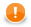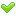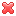### Relational Operators

The following operators serve to compare some subexpressions when you want to obtain a boolean value result. Each of the mentioned signs can be used. These signs can be used more times in one expression. In such a case you can express priority of comparisons by parentheses.Important

If you choose the `.operator.` syntax, operator must be surrounded by white spaces. Example syntax for the `eq` operator:

CodeWorking?
5 .eq. 35 == 35 eq 35.eq(3)• Greater than

Each of the two signs below can be used to compare expressions consisting of numeric, date and string data types. Both data types in the expressions must be comparable. The result can depend on the order of the two expressions if they are of different data types.

• `>`
• `.gt.`
```boolean a = 4 > 3;
a = "dog" > "cat";
if ( date1 > date2 ) {}```
• Greater than or equal to

Each of the three signs below can be used to compare expressions consisting of the numeric, date and string data types. Both data types in the expressions must be comparable. The result can depend on the order of the two expressions if they are of different data types.

• `>=`
• `=>`
• `.ge.`
```boolean a = 3.5 >= 3.5;
a = "ls" >= "lsof";
a = date1 >= date2;```
• Less than

Each of the two signs below can be used to compare expressions consisting of numeric, date and string data types. Both data types in the expressions must be comparable. The result can depend on the order of the two expressions if they are of different data types.

• `<`
• `.lt.`
• Less than or equal to

Each of the three signs below can be used to compare expressions consisting of the numeric, date and string data types. Both data types in the expressions must be comparable. The result can depend on the order of the two expressions if they are of different data types.

• `<=`
• `=<`
• `.le.`
```int a = 7L < 8L;
if ( "awk" < "java" ) {}
a = date1 < date2;```
• Equal to

Each of the two signs below can be used to compare expressions of any data type. Both data types in the expressions must be comparable. The result can depend on the order of the two expressions if they are of different data types.

• `==`
• `.eq.`
`if( 5 == 5 ) {}`
• Not equal to

Each of the three signs below can be used to compare expressions of any data type. Both data types in the expressions must be comparable. The result can depend on the order of the two expressions if they are of different data types.

• `!=`
• `<>`
• `.ne.`
`if ( 9 != 8 ) {}`
• Matches regular expression

The operator serves to compare string and some regular expression. It returns `true`, if the whole string matches the regular expression, otherwise returns `false`. If the right operand is `null`, operator fails.

`boolean b = "cat" ~= "[a-z]{3}"; `
• `~=`
• `.regex.`
```boolean b1 = "new bookcase" ~= ".*book.*";  // true
boolean b2 = "new bookcase" ~= "book";      // false
boolean b3 = "new bookcase" ~= null;        // fails```
• Contains regular expression

The operator serves to compare string and some regular expression. It returns `true`, if the string contains a substring that matches the regular expression, otherwise returns `false`.

• `?=`
`boolean b = "miredo" ?= "redo";`